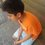# How can it not exist?

How do you define "Undefined"?

Does it mean that an expression has many different values?

Is $\sqrt { -1 }$ undefined?

Is ${ 0 }^{ 0 }$ undefined?

But the main question is:-

$Is\quad { 0 }^{ { 0 }^{ ...........\infty \quad times } }\quad undefined?\quad Or\quad it\quad doesn't\quad exist?$

One way to solve it:-

$x={ 0 }^{ { 0 }^{ ...........\infty \quad times } }\\ \therefore x={ 0 }^{ x }\quad \\ If\quad we\quad take\quad x\quad as\quad zero,\quad we\quad get,\\ 0={ 0 }^{ 0 }\quad which\quad is\quad not\quad true.\\ If\quad we\quad take\quad x\quad as\quad n,\quad where\quad n\neq 0,\\ we\quad get\quad n={ 0 }^{ n }.\\ \therefore n=0,\quad which\quad is\quad not\quad true.\\ \\ So\quad this\quad proves\quad that\quad { 0 }^{ { 0 }^{ ...........\infty \quad times } }\quad is\quad not\\ undefined,\quad but\quad it\quad doesn't\quad exist.\\ \\ \\ \$/extract_itex] How is it possible that a given value doesn't exist? There are 4 types of answers to all mathematical questions:- 1) Finite 2) Infinite 3) Undefined 4) Imaginary So how is it possible that it doesn't exist? Can you give any more examples of expressions whose value doesn't exist? Can you say that this is undefined? Plot twist: Another way to solve:- $x={ 0 }^{ { 0 }^{ ...........\infty \quad times } }\\ \therefore { 0 }^{ x }={ 0 }^{ { 0 }^{ ...........\infty \quad times } }\\ \therefore { 0 }^{ x }={ 0 }^{ x }\\ All\quad values\quad of\quad x\quad except\quad 0\quad satisfy\\ this.\quad So\quad x\quad is\quad undefined.\\ \\ \\ \\ \\ \\$ Now we have 2 values of x-> Undefined and doesn't exist. Is there anyone who can clear my confusion? I request you all to give your views and solutions in the comment box. I will be highly obliged.Note by Archit Boobna 6 years, 8 months ago This discussion board is a place to discuss our Daily Challenges and the math and science related to those challenges. Explanations are more than just a solution — they should explain the steps and thinking strategies that you used to obtain the solution. Comments should further the discussion of math and science. When posting on Brilliant: • Use the emojis to react to an explanation, whether you're congratulating a job well done , or just really confused . • Ask specific questions about the challenge or the steps in somebody's explanation. Well-posed questions can add a lot to the discussion, but posting "I don't understand!" doesn't help anyone. • Try to contribute something new to the discussion, whether it is an extension, generalization or other idea related to the challenge. • Stay on topic — we're all here to learn more about math and science, not to hear about your favorite get-rich-quick scheme or current world events. MarkdownAppears as *italics* or _italics_ italics **bold** or __bold__ bold - bulleted- list • bulleted • list 1. numbered2. list 1. numbered 2. list Note: you must add a full line of space before and after lists for them to show up correctly paragraph 1paragraph 2 paragraph 1 paragraph 2 [example link](https://brilliant.org)example link > This is a quote This is a quote  # I indented these lines # 4 spaces, and now they show # up as a code block. print "hello world" # I indented these lines # 4 spaces, and now they show # up as a code block. print "hello world" MathAppears as Remember to wrap math in $$ ... $$ or \[ ... $ to ensure proper formatting.
2 \times 3 $2 \times 3$
2^{34} $2^{34}$
a_{i-1} $a_{i-1}$
\frac{2}{3} $\frac{2}{3}$
\sqrt{2} $\sqrt{2}$
\sum_{i=1}^3 $\sum_{i=1}^3$
\sin \theta $\sin \theta$
\boxed{123} $\boxed{123}$

## Comments

Sort by:

Top Newest

It is Undefined..check it out below

There are 7 indeterminant forms namely,

$\frac{0}{0}, \frac{\infty}{\infty}, \infty - \infty, \infty \times 0, \infty^{0}, 0^{0}, 1^{\infty}$

here $0$, $1$ and $\infty$ are tending $0$ and tending $\infty$

But

$\frac{exact 0}{exact 0}, \frac{tending0}{exact 0}, \infty + \infty, \infty \times \infty, \infty^{\infty}, \pm \infty$

Are undefined

Let me explain further

$exact 1^{\infty} = 1$

But

$tending 1 ^{\infty}$ is indeterminant

Another example

$\frac{exact 0}{tending 0} = 0$

But

$\frac{tending 0}{tending 0}$ is indeterminant

- 6 years, 8 months ago

Log in to reply

Thank you so much for the explanation

- 6 years, 8 months ago

Log in to reply

You missed indeterminant form...

- 6 years, 8 months ago

Log in to reply

Thanks. But please tell me more about indeterminant form.

- 6 years, 8 months ago

Log in to reply

Is there any difference between indeterminant and undefined?

- 6 years, 8 months ago

Log in to reply

{ y }^{ x{ y }^{ y } }

- 6 years, 8 months ago

Log in to reply

kuch bhi

- 6 years, 8 months ago

Log in to reply

hmmmmmmmm

- 6 years, 8 months ago

Log in to reply

×

Problem Loading...

Note Loading...

Set Loading...